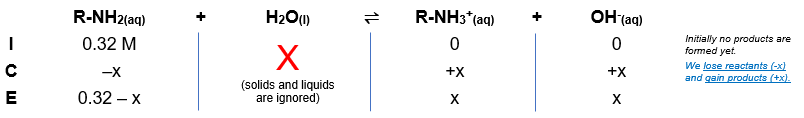# Problem: What is the pH of 0.32 M R-NH2 (amine - a weak base) with a Kb of 6.43x10-6 at 21°C? Enter pH to 2 decimal places.

###### FREE Expert Solution

Remember that weak bases partially dissociate in water and that bases accept H+ from the acid (water in this case). The dissociation of R-NH2 is as follows:

Equilibrium reaction:        R-NH2(aq) + H2O(l)  R-NH3+(aq) + OH-(aq)

Step 1: Construct an ICE chart for the reaction.Step 2: Write the Kb expression and calculate for Kb.

$\overline{){{\mathbf{K}}}_{{\mathbf{b}}}{\mathbf{=}}\frac{\mathbf{products}}{\mathbf{reactants}}}\phantom{\rule{0ex}{0ex}}{\mathbf{K}}_{\mathbf{b}}\mathbf{=}\frac{\mathbf{\left[}\mathbf{R}\mathbf{-}{{\mathbf{NH}}_{\mathbf{3}}}^{\mathbf{+}}\mathbf{\right]}\mathbf{\left[}{\mathbf{OH}}^{\mathbf{-}}\mathbf{\right]}}{\left[R-{\mathrm{NH}}_{2}\right]}$

Solids and liquids are not included in the expression

Step 3: Calculate the equilibrium concentrations.

100% (100 ratings)###### Problem Details

What is the pH of 0.32 M R-NH2 (amine - a weak base) with a Kb of 6.43x10-6 at 21°C? Enter pH to 2 decimal places.